# Practice Questions with Solutions: Factors and Multiples Notes | Study Mathematics for Class 4 - Class 4

## Class 4: Practice Questions with Solutions: Factors and Multiples Notes | Study Mathematics for Class 4 - Class 4

The document Practice Questions with Solutions: Factors and Multiples Notes | Study Mathematics for Class 4 - Class 4 is a part of the Class 4 Course Mathematics for Class 4.
All you need of Class 4 at this link: Class 4

Question 1: Find first four multiples of 6.

We know,
6 x 1 = 6, 6 x 2 = 12,
6 X 3 = 18, 6 X 4 = 24

Hence, the first 4 multiples of 6 are 6, 12, 18 and 24.

Question 2: Is 8 a factor of 112?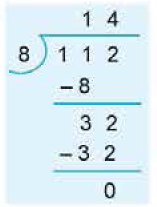Here 8 divides 112 exactly, so 8 is a factor of 112.

Question 3: In the following identify prime numbers and composite numbers.
12     15     19     23     27     51     69     83

12 = 1 x 12, 12 = 2 x 6, 12 = 3 x 4,

All the factors of 12 are 1, 2, 3, 4, 6 and 12.
15 = 1 x 15, 15 = 3 x 5.

All the factors of 15 are 1, 3, 5 and 15
19 = 1 x 19

Question 4: Express 420 as the product of prime numbers.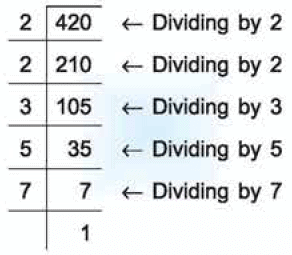Hence, 420 = 2 x 2 x 3 x 5 x 7.
This process can be shown diagramatically, known as factor tree.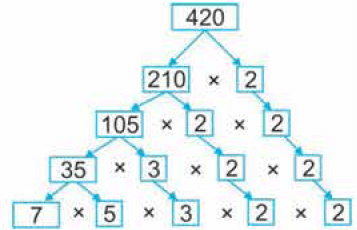Question 5: Find all the common factors of :
(a) 24 and 40
(b) 20 and 64

(a) 24 = 1 x 24, 24 = 2 x 12, 24 = 3 x 8, 24 = 4 x 6
Therefore, all the factors of 24 are 1, 2, 3, 4, 6, 8, 12 and 24.
Also, 40 = 1 x 40, 40 = 2 x 20, 40 = 4 x 10, 40 = 5 x 8.
Therefore, all the factors of 40 are 1, 2, 4, 5, 8, 10, 20 and 40.
Thus, common factors of 24 and 40 are 1, 2, 4 and 8.
(b) 20 = 1 x 20, 20 = 2 x 10 1 20 = 4 x 5
Therefore, all the factors of 20 are 1, 2, 4, 5, 10 and 20.
Also, 64 = 1 x 64, 64 = 2 x 32, 64 = 4 x 16, 64 = 8 x 8.
Therefore, all the factors of 64 are 1, 2, 4, 8, 16, 32 and 64.
Thus, common factors of 20 and 64 are 1, 2 and 4.

Question 6: Find the first two common multiples of : (a) 4 and 10 (b) 5, 6 and 15.

(a) Multiples of 4 are 4, 8, 12, 16, 20, 24, 28, 32, 36, 40, .....
Multiples of 10 are 10, 20, 30, 40, .....
Thus, the first two common multiples of 4 and 10  are 20 and 40.
(b) Multiples of 5 are 5, 10, 15, 20, 25, 30, 35, 40, 45, 60, 65, .....
Multiples of 6 are 6, 12, 18, 24, 30, 36, 42, 48, 54, 60, .....

Multiples of 15 are 15, 30, 45, 60, 75, .....
Thus, the first two common multiples of 5, 6 and 15 are 30 and 60.

Question 7: Find the L.C.M. of 12 and 18.

Multiples of 12 are 12, 24, 36, 48, 60, 72, .....
Multiples of 18 are 18, 36, 54, 72, 90, .....
Thus, common multiples of 12 and 18 are 36, 72, .....
Therefore, L.C.M. of 12 and 18 is 36.

Question 8: Write the first 6 multiples of 15.

We know, 15 X 1 = 15, 15 X 2 = 30
15 X 3 = 45, 15 X 4 = 60
15 X 5 75, 15 X 6 = 90
Hence, the first 6 multiples of 15 are 15, 30, 45, 60, 75 and 90.

Question 9: Is 9 a factor of 1, 854?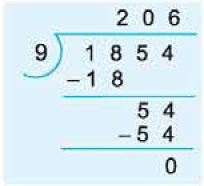Here 9 divides 1, 854 exactly, so 9 is a factor of 1, 854.

Question 10: Draw a factor tree to show the prime factorization of 42, 96 and 108.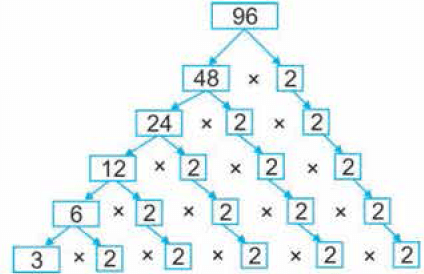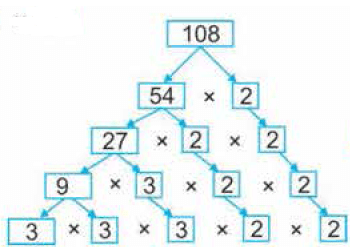Question 11: Find the H.C.F. of 25 and 45.

25 = 1 x 25, 25 = 5 x 5
All the factors of 25 are 1, 5 and 25.
Also, 45 = 1 x 45, 45 = 3 x 15, 45 = 5 x 9.
All the factors of 45 are 1, 3, 5, 9, 15 and 45.
Common factors of 25 and 45 are 1 and 5.
Thus, H.C.F. of 25 and 45 is 5.

Question 12: Find the LCM of 24 and 30 by division method.

LCM of 24 and 30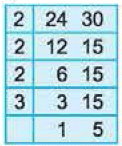= 2 x 2 x 2 x 3 x 5 = 120.

Question 13: Is 108 a multiple of 9?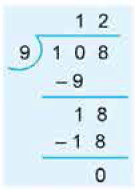Here, 9 divides 108 exactly.
Thus, 108 is a multiple of 9.

Question 14: Is 8 a factor of 1, 625?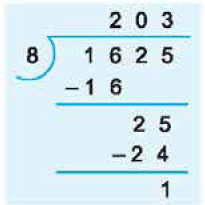Here 8 does not divide 1, 625 exactly, so 8 is not a factor of 1, 625.

Question 15: Find the H.C.F. of 18, 45 and 63.

18 = 1 x 18, 18 = 2 x 9, 18 = 3 x 6

All the factors of 18 are 1, 2, 3, 6, 9 and 18.
Also, 45 = 1 x 45, 45 = 3 x 15, 45 = 5 x 9.
All the factors of 45 are 1, 3, 5, 9, 15 and 45.
Also, 63 = 1 x 63, 63 = 3 x 21 , 63 = 7 x 9.
All the factors of 63 are 1, 3, 7, 9, 21 and 63.
Common factors of 18, 45 and 63 are 1, 3 and 9.
Thus, H.C.F. of 18, 45 and 63 is 9.

Question 16: Find the H.C.F. of 15 and 28.

15 = 1 X 15, 15 = 3 X 5.

All the factors of 15 are 1, 3, 5 and 15.
Also, 28 = 28 x 1, 28 = 2 x 14, 28 = 4 x 7
All the factors of 28 are 1, 2, 4, 7, 14 and 28.
Common factor of 15 and 28 is 1.
Thus, H.C.F. of 15 and 28 is 1.

Question 17: Find all the factors of 18.

18 = 1 x 18
18 = 2 X 9

18 = 3 X 6
Hence, all the factors of 18 are 1,  2, 3, 6, 9 and 18.

Question 18: Is 116 a multiple of 8?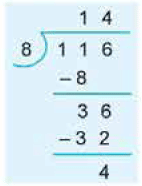Here, 8 does not divide 116 exactly.
Thus, 116 is not a multiple of 8.

Question 19: Find the LCM of 18, 36 and 42 by long division method.

LCM of 18, 36 and 42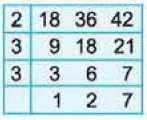= 2 x 3 x 3 x 2 x 7 : 252.

Question 20: Find the 8th multiple of 8?

8th multiple of 12 = 12 x 8 = 96.

Question 21: Find all the factors of 45.

45 = 1 x 45
45 = 3 X 15

45 = 5 X 9
Hence, all the factors of 45 are 1, 3, 5, 9, 15 and 45

Question 22: Find the H.C.F. of 20 and 36.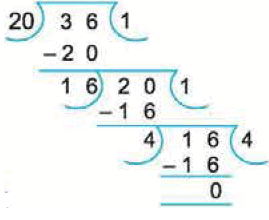We have :
Here, the last divisor is 4.
Hence, the H.C.F. of 20 and 36 is 4.

Question 23: Find the H.C.F. of 42 and 78 .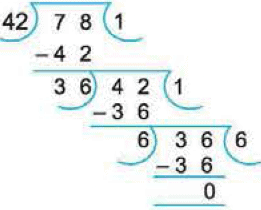We have:
Here, the last divisor is 6.
Hence, the H.C.F. of 42 and 78 is 6.

Question 24: Find the H.C.F. of 20, 35 and 50.

First we find the H.C.F. of any two numbers (say 20 and 35).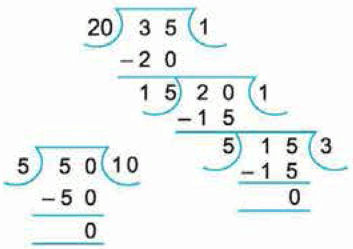Since, the last divisor is 5, So, 5 is the HCF of 20 and 35.
Now, we find the HCF of 5 and 50. Hence, H.C.F. of 20, 35 and 50 is 5.

The document Practice Questions with Solutions: Factors and Multiples Notes | Study Mathematics for Class 4 - Class 4 is a part of the Class 4 Course Mathematics for Class 4.
All you need of Class 4 at this link: Class 4Use Code STAYHOME200 and get INR 200 additional OFF

## Mathematics for Class 4

15 videos|68 docs|32 tests

Track your progress, build streaks, highlight & save important lessons and more!

,

,

,

,

,

,

,

,

,

,

,

,

,

,

,

,

,

,

,

,

,

;